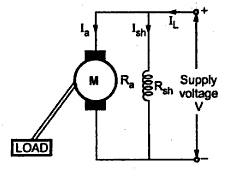### D.C. Shunt Motor

In this type, the field winding is connected across the armature winding and the combination is connected across the supply, as shown in the Fig. 1.Fig. 1 D.C. shunt motor

Let Rsh be the resistance of shunt field winding.
Ra be the resistance of armature winding.
The value of Ra is very small while Rsh is quite large. Hence shunt field winding has more number of turns with less cross-sectional area.
1.1 Voltage and Current Relationship
The voltage across armature and field winding is same equal to the supply voltage V.
The total current drawn from the supply is denoted as line current IL.
IL = Ia + Ish
Ish = V/Rsh
and            V = E + Ia Ra + Vbrush
Vbrush is generally neglected.
Now flux produced by the field winding is proportional to the current passing through it i.e. Ish.

Note : As long as supply voltage is constant, which is generally so in practice, the flux produced is constant. Hence d.c. shunt motor is called constant flux motor.

Related Articles :